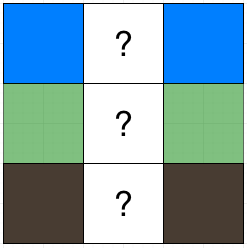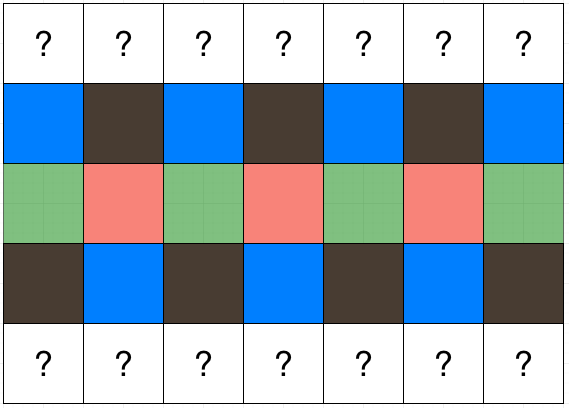Consider a table of $m\times n$ cells, which are colored by four colors Azure, Coral, Green, and Taupe. The only condition for it to be nice is that every subtable of $2\times 2$ has 4 distinct colors. (1)

Given a colored table, we need to re-color it so that it satisfies the condition above. What is the minimum number of cells that need to be re-colored? Read the problem statement.

We need to prove the following theorem:

Theorem. Such a table satisfying (1) has:

• every row has two alternate colors, or
• every column has two alternate colors

Proof

It is obvious that every row or column cannot have only one colors. We will prove that if a column of the nice table contains at least 3 different colors, then every row contains only 2 colors.

Suppose that column $j$ has at least 3 different colors. We can find $i$ such that the colors of $a[i][j]$ (i-th row, j-th column), $a[i-1][j]$, and $a[i+1][j]$ are pairwise different. Consider a column that is two columns away from $j$. Without loss of generosity, let it be the column $j+2$. Since $a$ is a nice table, $\{a[i][j], a[i-1][j], a[i][j+1], a[i-1][j+1]\}$ forms a full set of colors ACGT, and so is $\{a[i][j+1], a[i-1][j+1], a[i][j+2], a[i-1][j+2]\}$.

That implies $\{a[i][j], a[i-1][j]\} = \{a[i][j+2], a[i-1][j+2]\}$. Similarly we can show that $\{a[i][j], a[i+1][j]\} = \{a[i][j+2], a[i+1][j+2]\}$. The two equalities give us $a[i-1][j] = a[i-1][j+2], a[i][j] = a[i][j+2],$ and $a[i+1][j] = a[i+1][j+2]$.Columns are repeated

Until now, we’ve discovered that if there are three consecutive cells with different colors on column $j$, then the corresponding cells on column $j+2$ will have the same color in that order. Using induction we can prove that the corresponding cells on any column $k\equiv j\ (\mod 2)$ will have the same color in that order.

Furthermore, there is only one way to color three cells between column $j$ and $j+2$. This implies that we can figure out the color of the whole row $i-1$, $i$, and $i+1$.All cells on the rows can be deduced, and in each row the colors are alternating

We observe that in a nice table, if one row has two alternating colors, say A and C, then the nearby row also has two alternating colors (G and T), and all other rows also have two alternating colors (A-C or G-T). (QED)

A similar proof can be done to show the rest of the theorem.

Algorithm

Let $a$ be the nice table deduced from the given table through the minimum number of recoloring steps.

We shall check two cases: $a$ has rows of alternating colors, or $a$ has columns of alternating colors. Whichever has smaller amount of differences from the given table will be chosen.

If $a$ has rows of alternating colors, we select two colors for the first row, say X and Y, then the other two colors (say Z and W) will present in the second row, and the third row will have X and Y again, and so on.

But should the row begin with “XYXY…” or “YXYX…”? Greedily speaking, we should choose the pattern that minimize the number of differences, because our decision of which pattern to use on this row doesn’t affect other rows. We try all cases of X and Y, then continue with the case of columns of alternating colors, to select the best table.

See my solution in C++.